# RD Sharma Solutions for Class 6 Maths Chapter 4: Operations on Whole Numbers Exercise 4.3

## RD Sharma Solutions for Class 6 Maths Exercise 4.3 PDF

The concepts explained in solutions are easy for the students to revise while learning. Students can use them as reference materials while solving problems based on the RD Sharma textbook. The problems are solved in a comprehensive manner in order to understand the methods used in the annual exam. The solutions can be used by the students to improve their problem-solving abilities and score well in the exam. By solving a huge number of problems, the students can self-analyse the areas which require more practice before the exam. RD Sharma Solutions for Class 6 Maths Chapter 4 Operations on Whole Numbers Exercise 4.3 are provided here.

## RD Sharma Solutions for Class 6 Maths Chapter 4: Operations on Whole Numbers Exercise 4.3 Download the PDF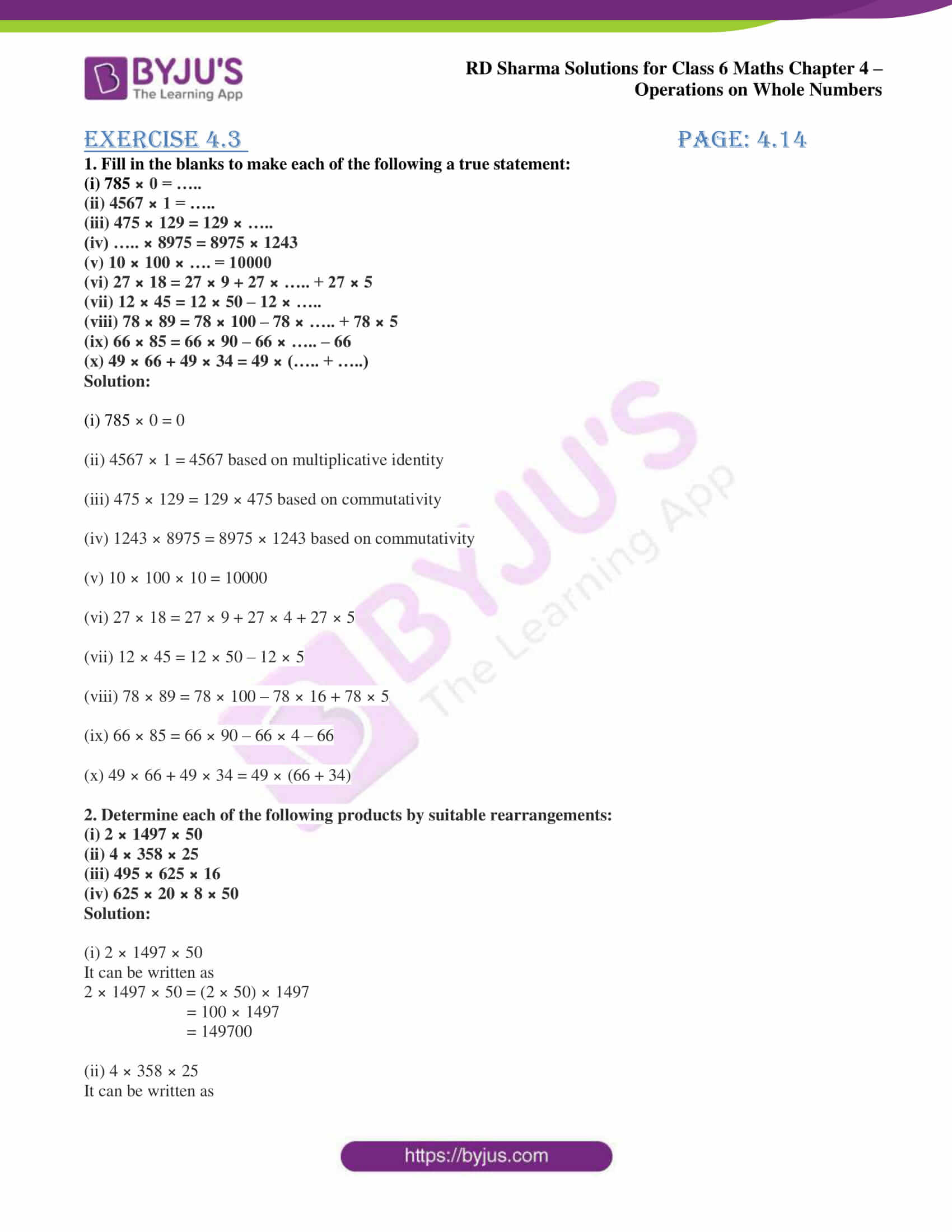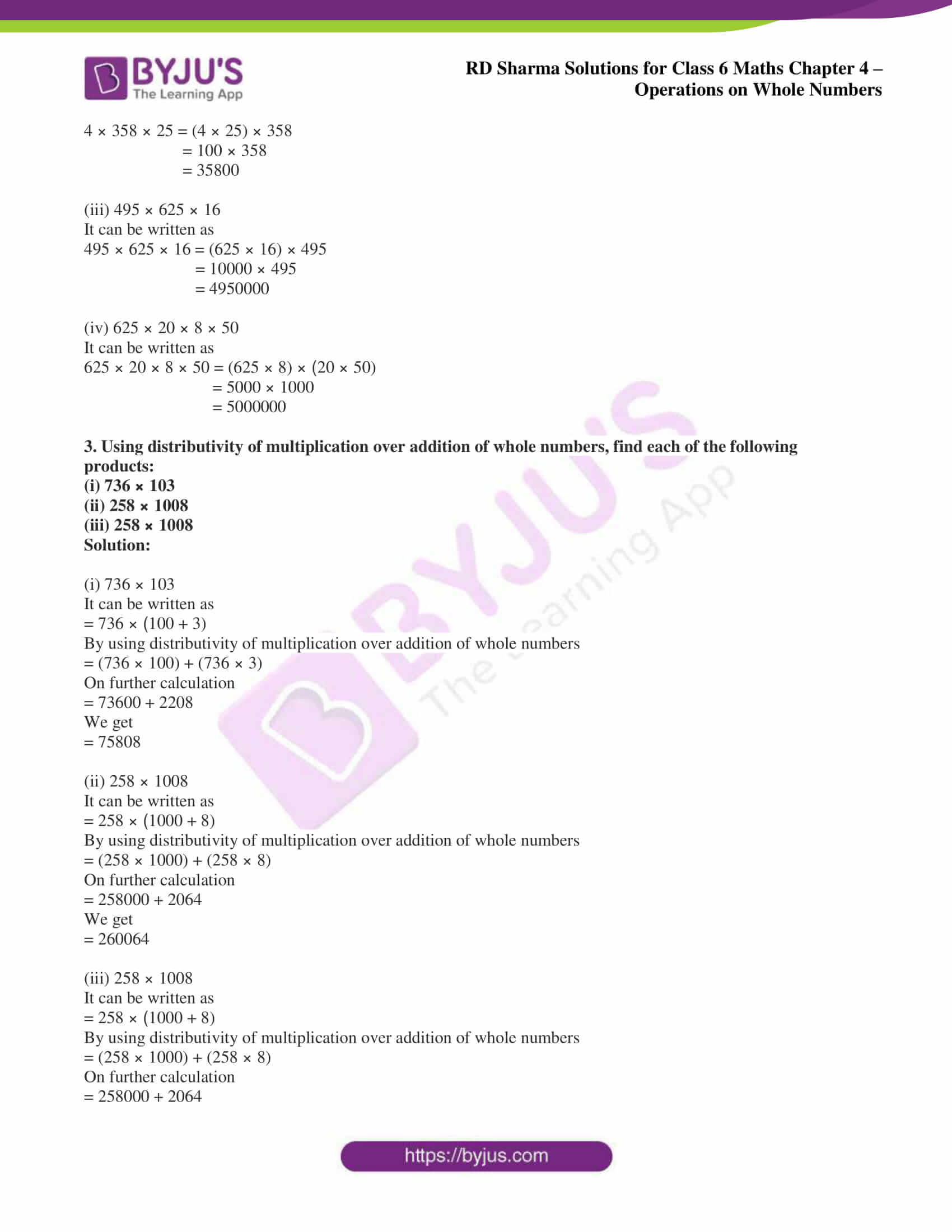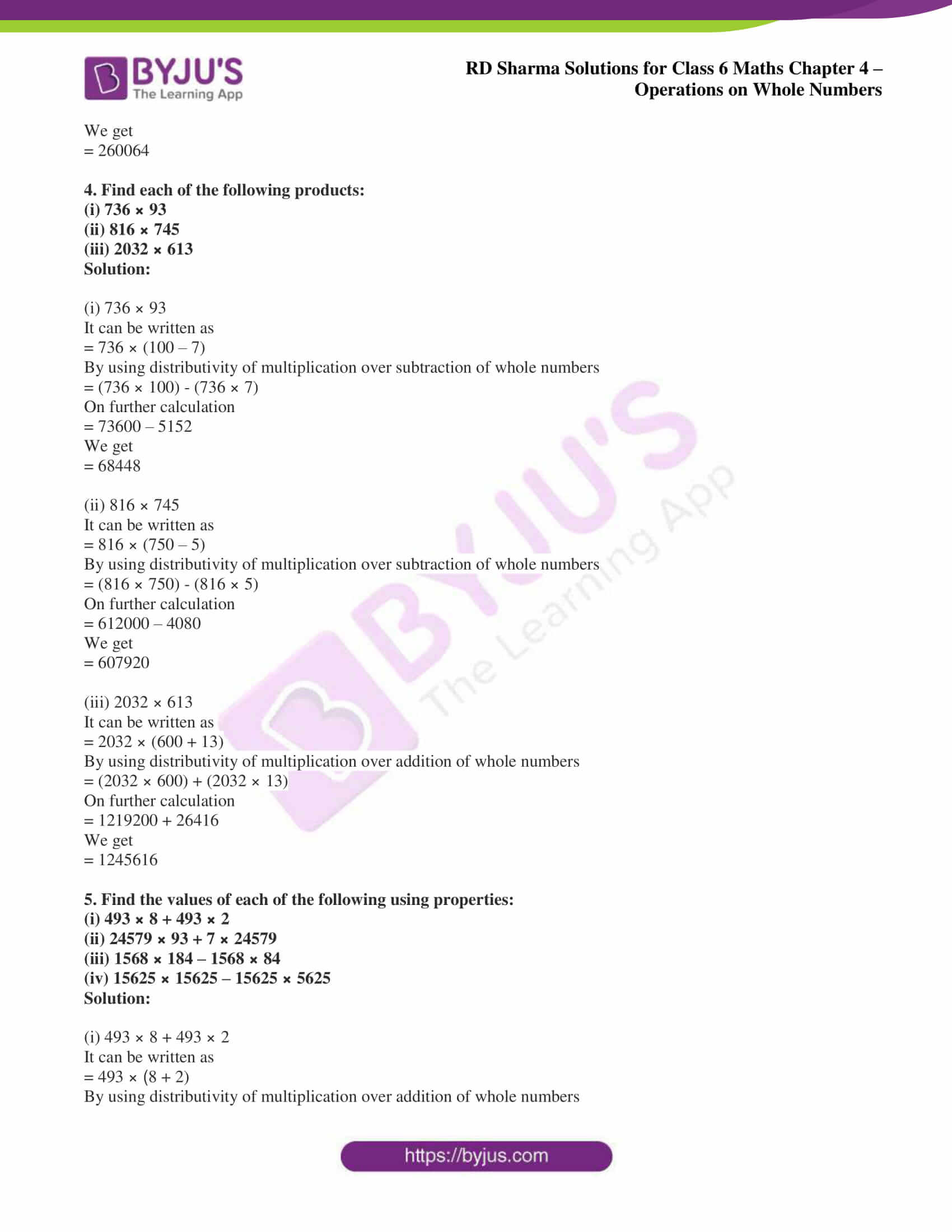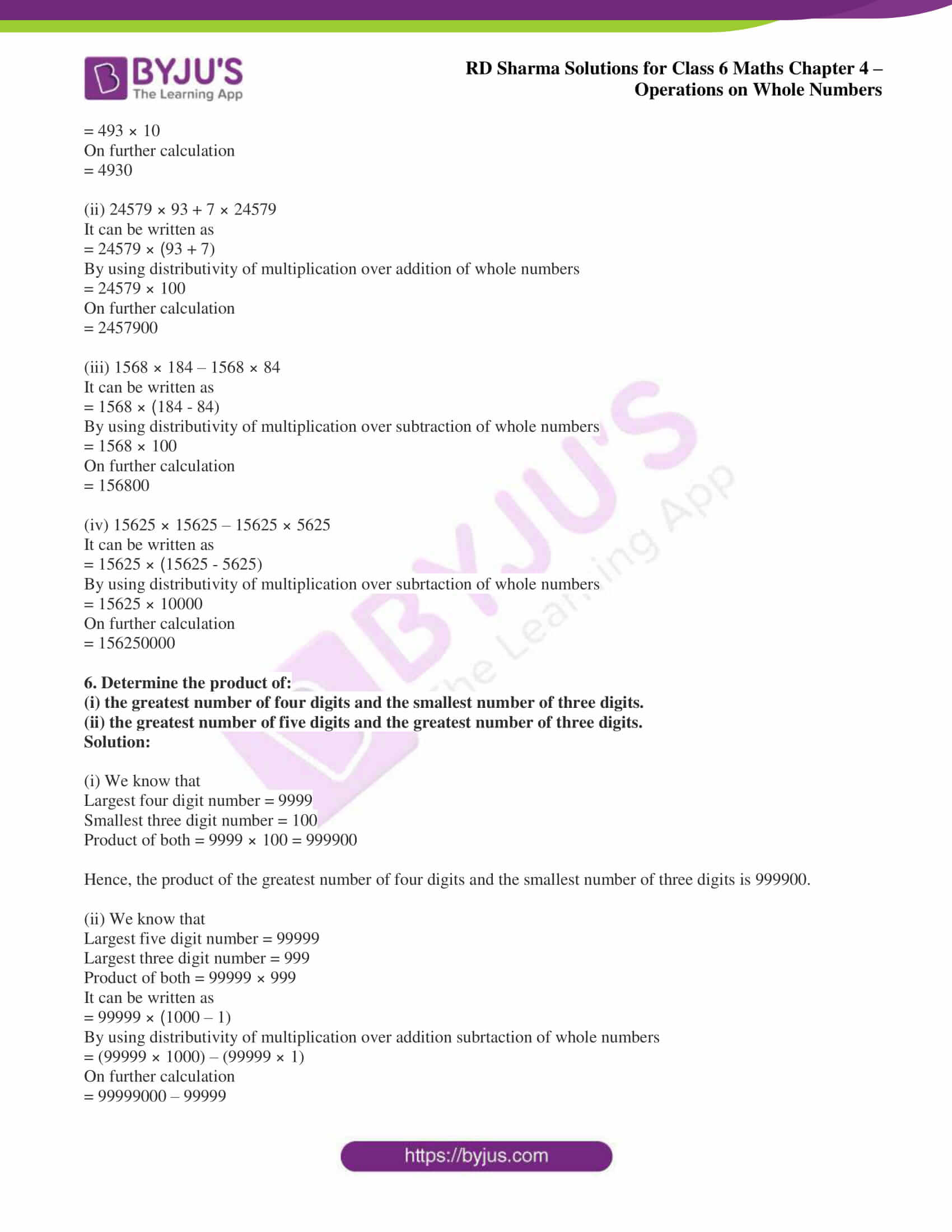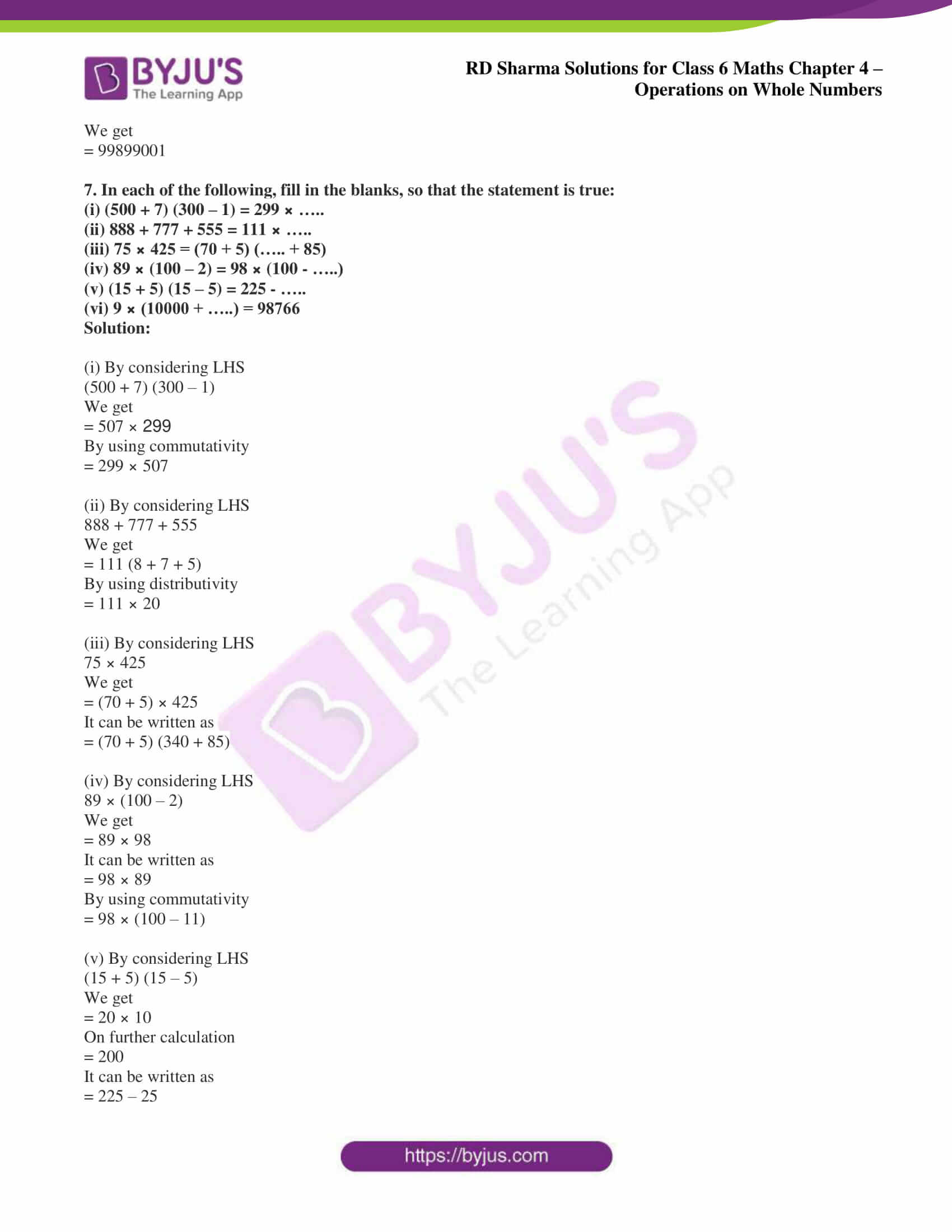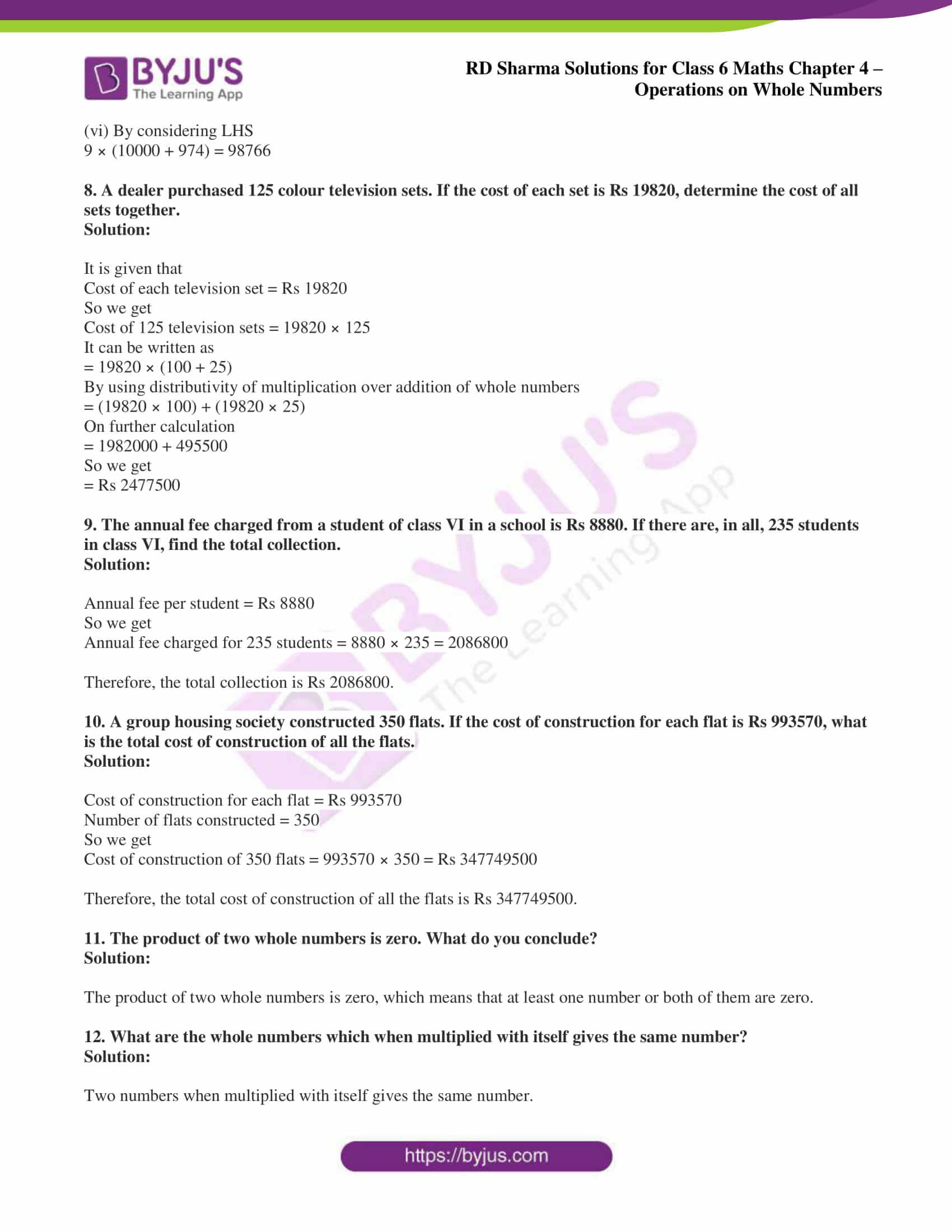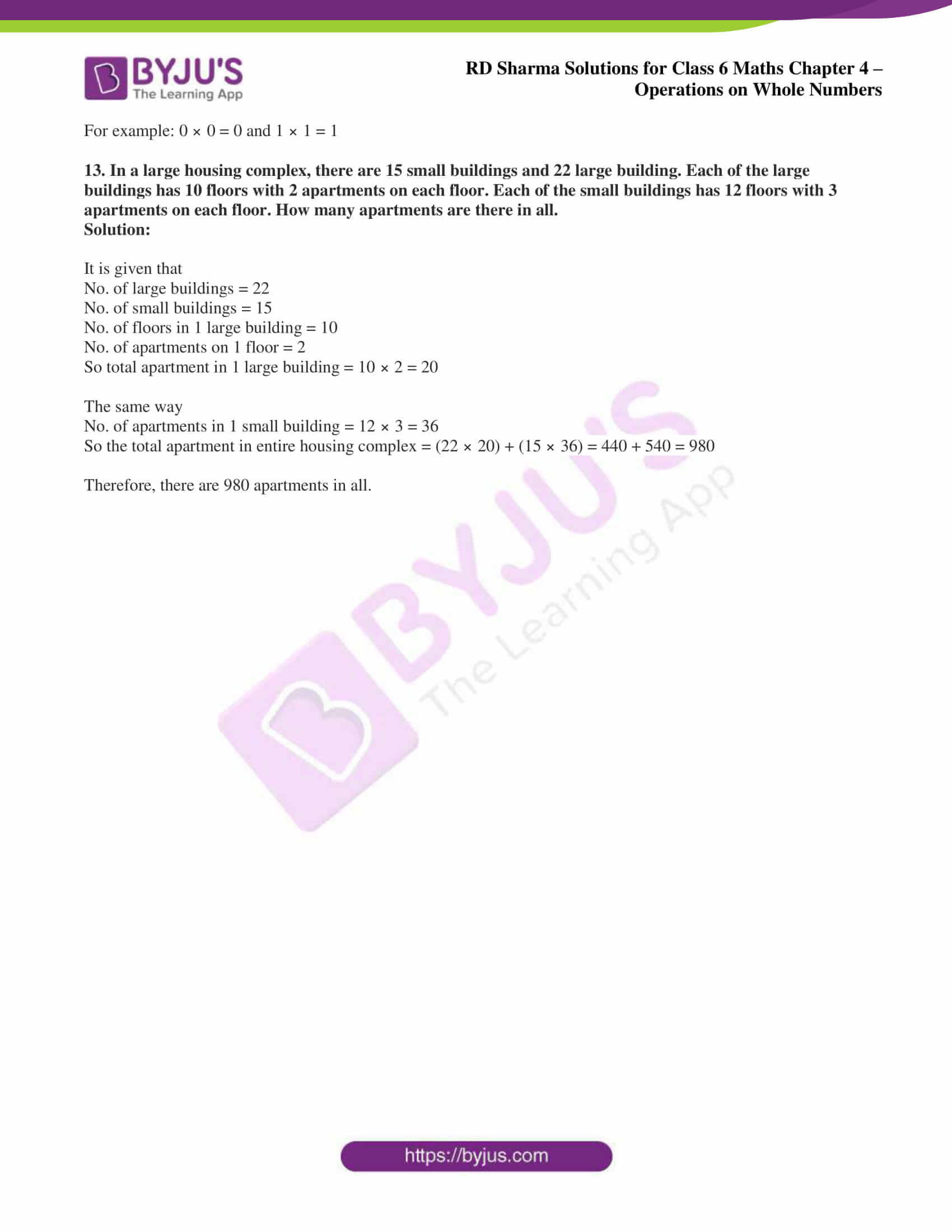## Access answers to Maths RD Sharma Solutions for Class 6 Chapter 4: Operations on Whole Numbers Exercise 4.3

1. Fill in the blanks to make each of the following a true statement:

(i) 785 × 0 = …..

(ii) 4567 × 1 = …..

(iii) 475 × 129 = 129 × …..

(iv) ….. × 8975 = 8975 × 1243

(v) 10 × 100 × …. = 10000

(vi) 27 × 18 = 27 × 9 + 27 × ….. + 27 × 5

(vii) 12 × 45 = 12 × 50 – 12 × …..

(viii) 78 × 89 = 78 × 100 – 78 × ….. + 78 × 5

(ix) 66 × 85 = 66 × 90 – 66 × ….. – 66

(x) 49 × 66 + 49 × 34 = 49 × (….. + …..)

Solution:

(i) 785 × 0 = 0

(ii) 4567 × 1 = 4567 based on multiplicative identity

(iii) 475 × 129 = 129 × 475 based on commutativity

(iv) 1243 × 8975 = 8975 × 1243 based on commutativity

(v) 10 × 100 × 10 = 10000

(vi) 27 × 18 = 27 × 9 + 27 × 4 + 27 × 5

(vii) 12 × 45 = 12 × 50 – 12 × 5

(viii) 78 × 89 = 78 × 100 – 78 × 16 + 78 × 5

(ix) 66 × 85 = 66 × 90 – 66 × 4 – 66

(x) 49 × 66 + 49 × 34 = 49 × (66 + 34)

2. Determine each of the following products by suitable rearrangements:

(i) 2 × 1497 × 50

(ii) 4 × 358 × 25

(iii) 495 × 625 × 16

(iv) 625 × 20 × 8 × 50

Solution:

(i) 2 × 1497 × 50

It can be written as

2 × 1497 × 50 = (2 × 50) × 1497

= 100 × 1497

= 149700

(ii) 4 × 358 × 25

It can be written as

4 × 358 × 25 = (4 × 25) × 358

= 100 × 358

= 35800

(iii) 495 × 625 × 16

It can be written as

495 × 625 × 16 = (625 × 16) × 495

= 10000 × 495

= 4950000

(iv) 625 × 20 × 8 × 50

It can be written as

625 × 20 × 8 × 50 = (625 × 8) × (20 × 50)

= 5000 × 1000

= 5000000

3. Using distributivity of multiplication over addition of whole numbers, find each of the following products:

(i) 736 × 103

(ii) 258 × 1008

(iii) 258 × 1008

Solution:

(i) 736 × 103

It can be written as

= 736 × (100 + 3)

By using distributivity of multiplication over addition of whole numbers

= (736 × 100) + (736 × 3)

On further calculation

= 73600 + 2208

We get

= 75808

(ii) 258 × 1008

It can be written as

= 258 × (1000 + 8)

By using distributivity of multiplication over addition of whole numbers

= (258 × 1000) + (258 × 8)

On further calculation

= 258000 + 2064

We get

= 260064

(iii) 258 × 1008

It can be written as

= 258 × (1000 + 8)

By using distributivity of multiplication over addition of whole numbers

= (258 × 1000) + (258 × 8)

On further calculation

= 258000 + 2064

We get

= 260064

4. Find each of the following products:

(i) 736 × 93

(ii) 816 × 745

(iii) 2032 × 613

Solution:

(i) 736 × 93

It can be written as

= 736 × (100 – 7)

By using distributivity of multiplication over subtraction of whole numbers

= (736 × 100) – (736 × 7)

On further calculation

= 73600 – 5152

We get

= 68448

(ii) 816 × 745

It can be written as

= 816 × (750 – 5)

By using distributivity of multiplication over subtraction of whole numbers

= (816 × 750) – (816 × 5)

On further calculation

= 612000 – 4080

We get

= 607920

(iii) 2032 × 613

It can be written as

= 2032 × (600 + 13)

By using distributivity of multiplication over addition of whole numbers

= (2032 × 600) + (2032 × 13)

On further calculation

= 1219200 + 26416

We get

= 1245616

5. Find the values of each of the following using properties:

(i) 493 × 8 + 493 × 2

(ii) 24579 × 93 + 7 × 24579

(iii) 1568 × 184 – 1568 × 84

(iv) 15625 × 15625 – 15625 × 5625

Solution:

(i) 493 × 8 + 493 × 2

It can be written as

= 493 × (8 + 2)

By using distributivity of multiplication over addition of whole numbers

= 493 × 10

On further calculation

= 4930

(ii) 24579 × 93 + 7 × 24579

It can be written as

= 24579 × (93 + 7)

By using distributivity of multiplication over addition of whole numbers

= 24579 × 100

On further calculation

= 2457900

(iii) 1568 × 184 – 1568 × 84

It can be written as

= 1568 × (184 – 84)

By using distributivity of multiplication over subtraction of whole numbers

= 1568 × 100

On further calculation

= 156800

(iv) 15625 × 15625 – 15625 × 5625

It can be written as

= 15625 × (15625 – 5625)

By using distributivity of multiplication over subrtaction of whole numbers

= 15625 × 10000

On further calculation

= 156250000

6. Determine the product of:

(i) the greatest number of four digits and the smallest number of three digits.

(ii) the greatest number of five digits and the greatest number of three digits.

Solution:

(i) We know that

Largest four digit number = 9999

Smallest three digit number = 100

Product of both = 9999 × 100 = 999900

Hence, the product of the greatest number of four digits and the smallest number of three digits is 999900.

(ii) We know that

Largest five digit number = 99999

Largest three digit number = 999

Product of both = 99999 × 999

It can be written as

= 99999 × (1000 – 1)

By using distributivity of multiplication over addition subrtaction of whole numbers

= (99999 × 1000) – (99999 × 1)

On further calculation

= 99999000 – 99999

We get

= 99899001

7. In each of the following, fill in the blanks, so that the statement is true:

(i) (500 + 7) (300 – 1) = 299 × …..

(ii) 888 + 777 + 555 = 111 × …..

(iii) 75 × 425 = (70 + 5) (….. + 85)

(iv) 89 × (100 – 2) = 98 × (100 – …..)

(v) (15 + 5) (15 – 5) = 225 – …..

(vi) 9 × (10000 + …..) = 98766

Solution:

(i) By considering LHS

(500 + 7) (300 – 1)

We get

= 507 × 299

By using commutativity

= 299 × 507

(ii) By considering LHS

888 + 777 + 555

We get

= 111 (8 + 7 + 5)

By using distributivity

= 111 × 20

(iii) By considering LHS

75 × 425

We get

= (70 + 5) × 425

It can be written as

= (70 + 5) (340 + 85)

(iv) By considering LHS

89 × (100 – 2)

We get

= 89 × 98

It can be written as

= 98 × 89

By using commutativity

= 98 × (100 – 11)

(v) By considering LHS

(15 + 5) (15 – 5)

We get

= 20 × 10

On further calculation

= 200

It can be written as

= 225 – 25

(vi) By considering LHS

9 × (10000 + 974) = 98766

8. A dealer purchased 125 colour television sets. If the cost of each set is Rs 19820, determine the cost of all sets together.

Solution:

It is given that

Cost of each television set = Rs 19820

So we get

Cost of 125 television sets = 19820 × 125

It can be written as

= 19820 × (100 + 25)

By using distributivity of multiplication over addition of whole numbers

= (19820 × 100) + (19820 × 25)

On further calculation

= 1982000 + 495500

So we get

= Rs 2477500

9. The annual fee charged from a student of class VI in a school is Rs 8880. If there are, in all, 235 students in class VI, find the total collection.

Solution:

Annual fee per student = Rs 8880

So we get

Annual fee charged for 235 students = 8880 × 235 = 2086800

Therefore, the total collection is Rs 2086800.

10. A group housing society constructed 350 flats. If the cost of construction for each flat is Rs 993570, what is the total cost of construction of all the flats.

Solution:

Cost of construction for each flat = Rs 993570

Number of flats constructed = 350

So we get

Cost of construction of 350 flats = 993570 × 350 = Rs 347749500

Therefore, the total cost of construction of all the flats is Rs 347749500.

11. The product of two whole numbers is zero. What do you conclude?

Solution:

The product of two whole numbers is zero, which means that at least one number or both of them are zero.

12. What are the whole numbers which when multiplied with itself gives the same number?

Solution:

Two numbers when multiplied with itself gives the same number.

For example: 0 × 0 = 0 and 1 × 1 = 1

13. In a large housing complex, there are 15 small buildings and 22 large building. Each of the large buildings has 10 floors with 2 apartments on each floor. Each of the small buildings has 12 floors with 3 apartments on each floor. How many apartments are there in all.

Solution:

It is given that

No. of large buildings = 22

No. of small buildings = 15

No. of floors in 1 large building = 10

No. of apartments on 1 floor = 2

So total apartment in 1 large building = 10 × 2 = 20

The same way

No. of apartments in 1 small building = 12 × 3 = 36

So the total apartment in entire housing complex = (22 × 20) + (15 × 36) = 440 + 540 = 980

Therefore, there are 980 apartments in all.

### RD Sharma Solutions for Class 6 Maths Chapter 4 – Operations on Whole Numbers Exercise 4.3

RD Sharma Solutions Class 6 Maths Chapter 4 Operations on Whole Numbers Exercise 4.3 have problems based on the properties of multiplication like closure property, commutativity, associativity and distributivity.

### Key Features of RD Sharma Solutions for Class 6 Maths Chapter 4: Operations on Whole Numbers Exercise 4.3

• Students can download exercise-wise solutions in PDF format and use them when they solve problems in the RD Sharma textbook.
• The students gain a clear idea about the concepts covered in each exercise.